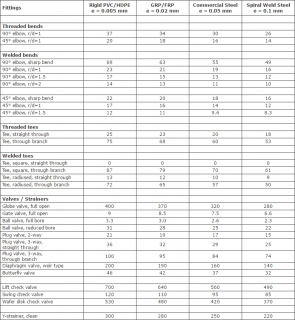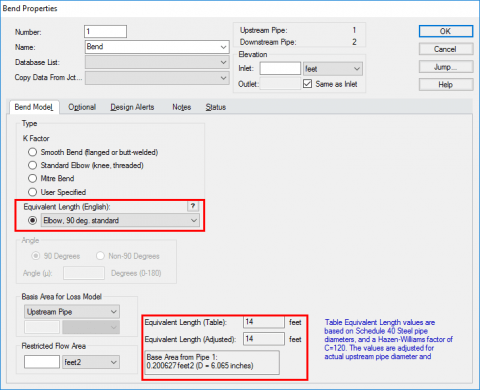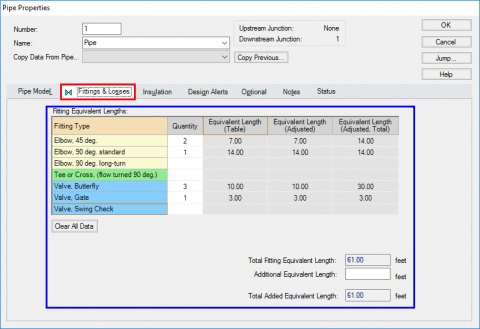One of the new features in AFT Fathom 9 was the ability to model equivalent lengths instead of K factors for various types of fittings.

Since AFT Fathom's existence, the standard K factor loss models have been used to quantify the pressure losses across a fitting such as an elbow, a valve, etc.  One of the reasons why the K factor method is very useful is because it is broad and applicable to a wide range of different fittings.  However, the equivalent length method is also a way that engineers will typically quantify the losses through their fittings and they will tack on the calculated equivalent length to the overall length of their piping itself.  Figure 1 below is a table of typical Equivalent Length values that you might want to use.Working with equivalent lengths in AFT Fathom is very easy and in order to use them, they simply need to be activated through the "Extended Options" in the Analysis menu.  Simply choose "Include Equivalent Lengths" and pick your desired units like in Figure 2 to turn on the equivalent length methods.After the Equivalent Lengths have been turned on, they can be modeled both with junctions and in the Pipe Fittings and Losses tab.  For junctions, simply open the property window for a valve or an elbow and then choose "Equivalent Length".  The appropriate equivalent length will be displayed and is calculated based upon the upstream pipe diameter, like that in Figure 3.As you can see in Figure 4, the Fittings and Losses tab in the Pipe Properties window is changed when the Equivalent Length options are turned on.  Simply specify the number of the different fitting types and the equivalent lengths will be calculated accordingly.Overall, that is all there is to it!  The Equivalent Length option will also make a fire protection analysis that adheres to NFPA codes much easier and more convenient.